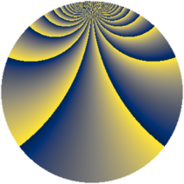# Properties

 Label 1148.2.caLevel $1148$ Weight $2$ Character orbit 1148.ca Rep. character $\chi_{1148}(15,\cdot)$ Character field $\Q(\zeta_{40})$ Dimension $2016$ Sturm bound $336$

# Learn more about

## Defining parameters

 Level: $$N$$ $$=$$ $$1148 = 2^{2} \cdot 7 \cdot 41$$ Weight: $$k$$ $$=$$ $$2$$ Character orbit: $$[\chi]$$ $$=$$ 1148.ca (of order $$40$$ and degree $$16$$) Character conductor: $$\operatorname{cond}(\chi)$$ $$=$$ $$164$$ Character field: $$\Q(\zeta_{40})$$ Sturm bound: $$336$$

## Dimensions

The following table gives the dimensions of various subspaces of $$M_{2}(1148, [\chi])$$.

Total New Old
Modular forms 2752 2016 736
Cusp forms 2624 2016 608
Eisenstein series 128 0 128

## Trace form

 $$2016q + 24q^{6} + 16q^{9} + O(q^{10})$$ $$2016q + 24q^{6} + 16q^{9} + 8q^{12} - 56q^{17} - 8q^{29} + 32q^{30} - 40q^{32} + 80q^{33} + 40q^{34} - 24q^{36} - 40q^{41} + 80q^{42} - 40q^{52} + 8q^{53} - 72q^{54} - 40q^{60} + 40q^{61} + 72q^{62} - 160q^{66} - 360q^{72} - 88q^{74} - 296q^{78} - 320q^{80} - 32q^{85} - 400q^{86} - 408q^{88} + 16q^{89} - 88q^{92} - 160q^{94} + 128q^{96} + 40q^{97} + O(q^{100})$$

## Decomposition of $$S_{2}^{\mathrm{new}}(1148, [\chi])$$ into newform subspaces

The newforms in this space have not yet been added to the LMFDB.

## Decomposition of $$S_{2}^{\mathrm{old}}(1148, [\chi])$$ into lower level spaces

$$S_{2}^{\mathrm{old}}(1148, [\chi]) \cong$$ $$S_{2}^{\mathrm{new}}(164, [\chi])$$$$^{\oplus 2}$$